##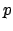-adic Numbers

This section is about the-adic numbers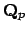, which are the completion of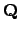with respect to the-adic valuation. Alternatively, to give a-adic integer in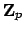is the same as giving for every prime power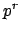an element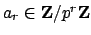such that if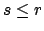then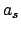is the reduction of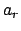modulo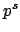. The fieldis then the field of fractions of.

We begin with the definition of the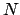-adic numbers for any positive integer. Section 16.2.1 is about the-adics in the special case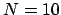; these are fun because they can be represented as decimal expansions that go off infinitely far to the left. Section 16.2.3 is about how the topology of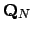is nothing like the topology of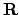. Finally, in Section 16.2.4 we state the Hasse-Minkowski theorem, which shows how to use-adic numbers to decide whether or not a quadratic equation in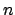variables has a rational zero.

Subsections
William Stein 2004-05-06